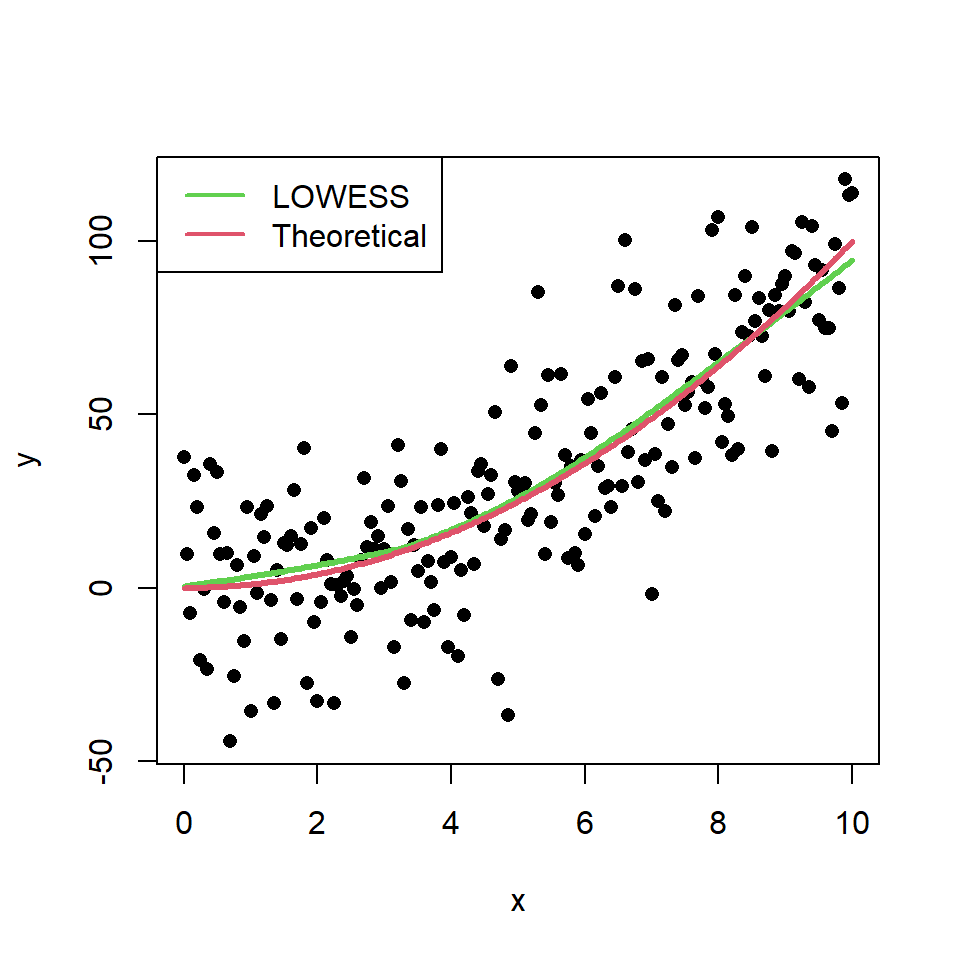# Scatter plot with regression line or curve in R

## Scatter plot based on a model

You can create a scatter plot based on a theoretical model and add it to the plot with the `lines` function. Consider the example of the following block of code as illustration.

``````# Data. Model: Y = X ^ 2
set.seed(54)
x <- seq(0, 10, by = 0.05)
y <- x ^ 2 + rnorm(length(x), sd = 20)

# Scatter plot and underlying model
plot(x, y, pch = 16)
lines(x, x ^ 2, col = 2, lwd = 3)

# Text
text(2, 70, expression(Y == X ^ 2))``````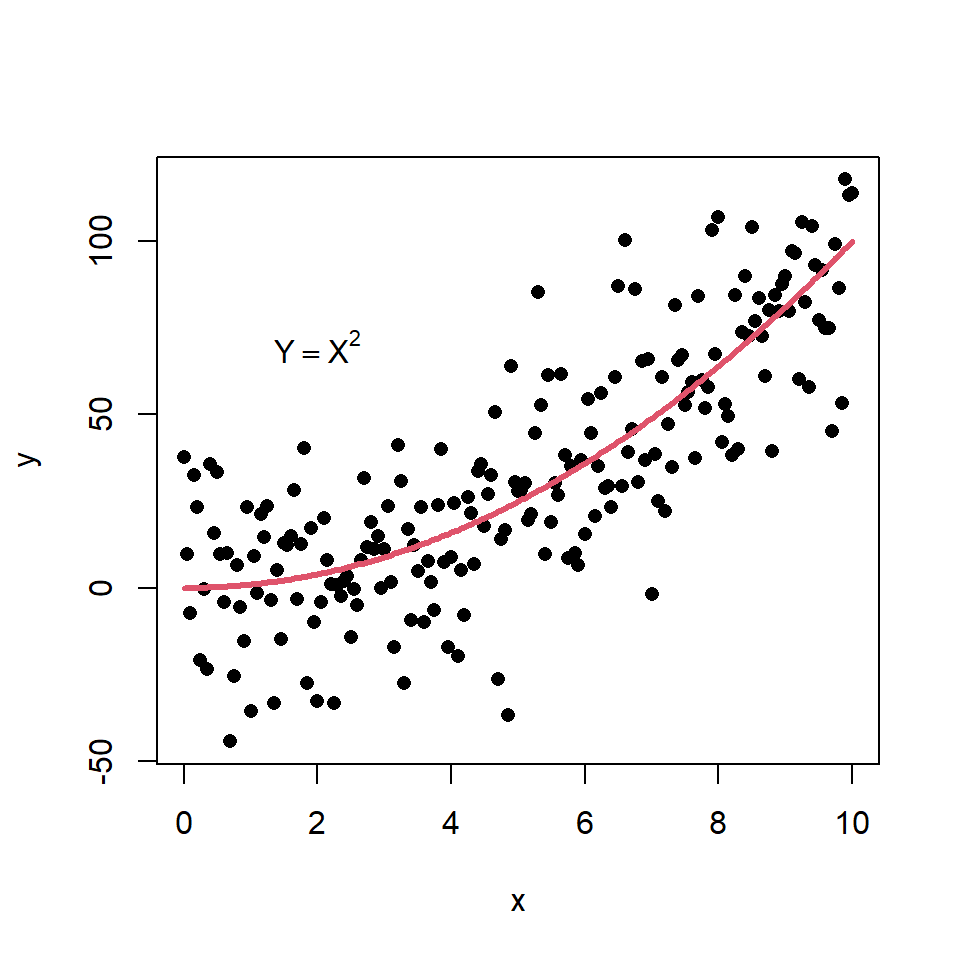## Scatter plot with linear regression

You can add a regression line to a scatter plot passing a `lm` object to the `abline` function. Recall that `coef` returns the coefficients of an estimated linear model.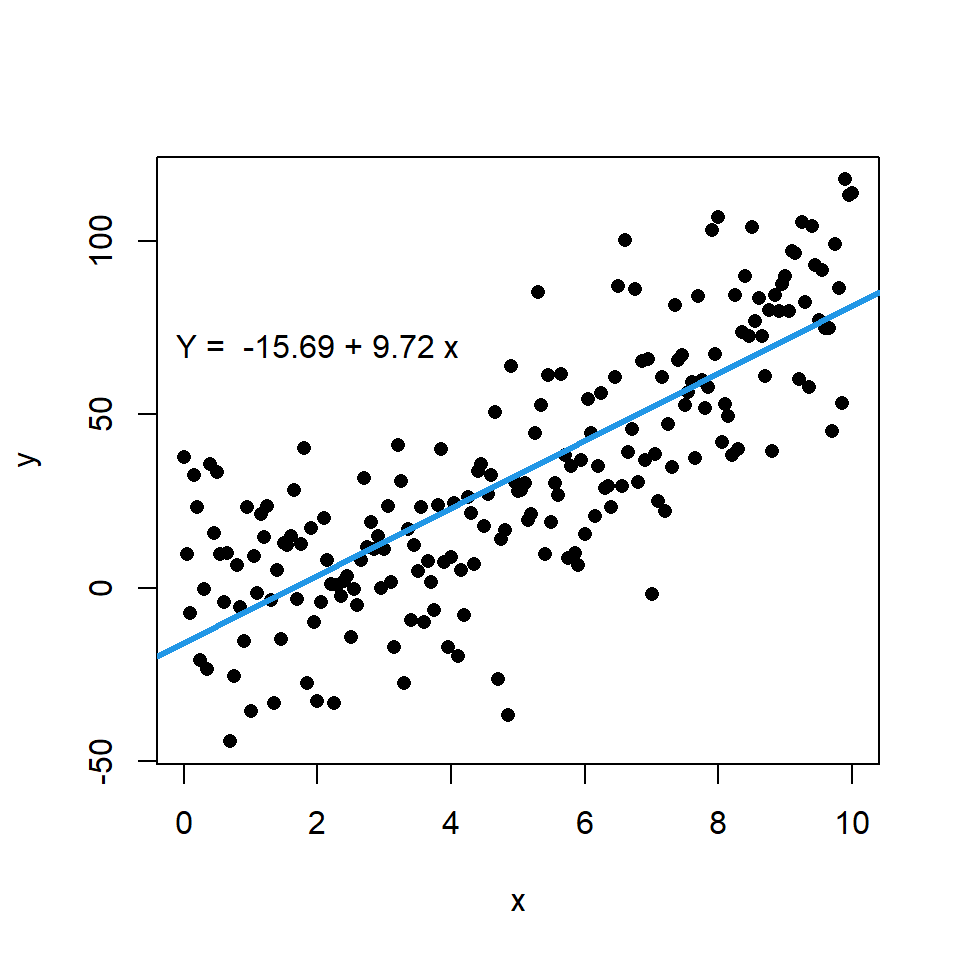``````# Data. Model: Y = X ^ 2
set.seed(54)
x <- seq(0, 10, by = 0.05)
y <- x ^ 2 + rnorm(length(x), sd = 20)

# Linear model
model <- lm(y ~ x)

# Scatter plot and linear regression line
plot(x, y, pch = 16)
abline(model, col = 4, lwd = 3)

# Text
coef <- round(coef(model), 2)
text(2, 70,  paste("Y = ", coef, "+", coef, "x"))``````

In addition, if you want you can display the error terms or residuals between actual and estimated values by using the `segments` function. For that purpose you can input the variable `x` to the `x0` and `x1` arguments, the variable `y` to `y0` and the estimate of the model, which is calculated with `predict`, to `y1`.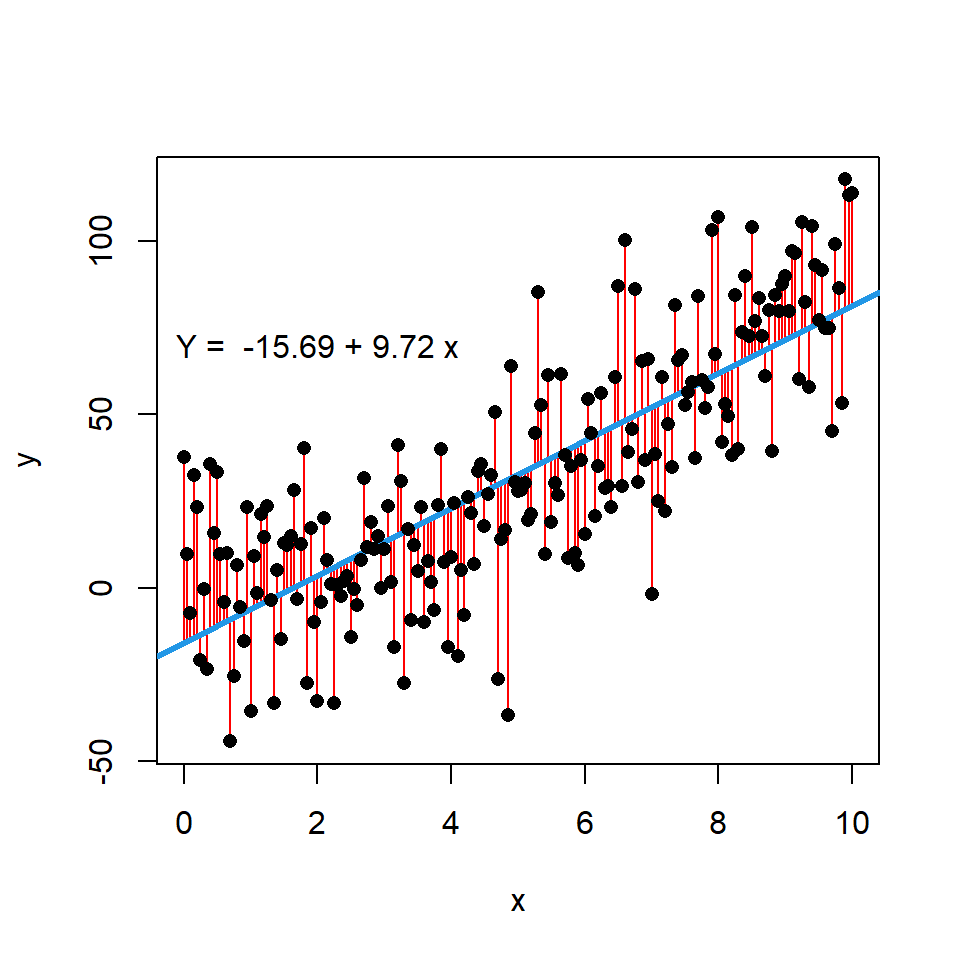``````# Data. Model: Y = X ^ 2
set.seed(54)
x <- seq(0, 10, by = 0.05)
y <- x ^ 2 + rnorm(length(x), sd = 20)

# Linear model
modelo <- lm(y ~ x)

# Scatter plot and linear regression line
plot(x, y, pch = 16)

# Segments with error terms
segments(x0 = x, x1 = x, y0 = y, y1 = predict(modelo),
lwd = 1, col = "red")

# Regression line
abline(modelo, col = 4, lwd = 3)

# Paint the points again over the segments
points(x, y, pch = 16)

# Text
coef <- round(coef(modelo), 2)
text(2, 70,  paste("Y = ", coef, "+", coef, "x")) ``````

## Scatter plot with LOWESS regression curve

The LOWESS smoother uses locally-weighted polynomial regression. This non-parametric regression can be estimated with `lowess` function.

``````# Data. Model: Y = X ^ 2
set.seed(54)
x <- seq(0, 10, by = 0.05)
y <- x ^ 2 + rnorm(length(x), sd = 20)

# Scatter plot and LOWESS regression curve
plot(x, y, pch = 16)
lines(lowess(x, y), col = 3, lwd = 3)``````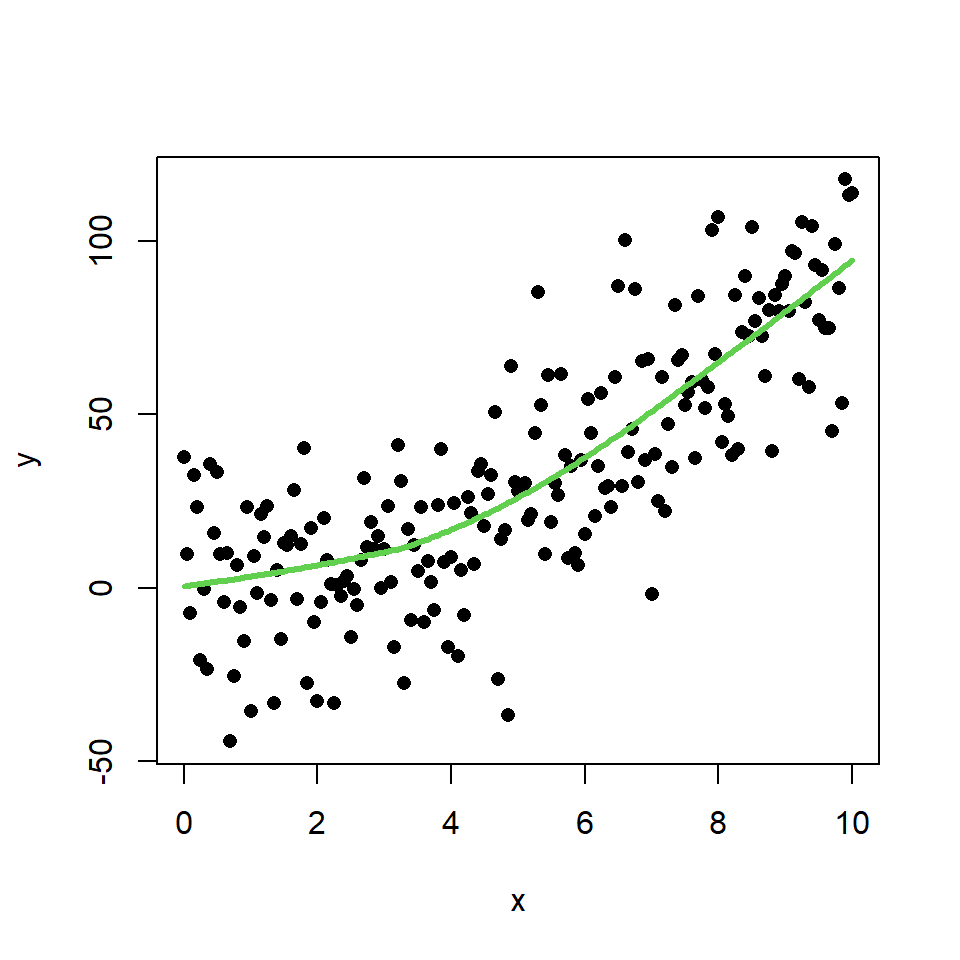In this scenario, this method makes a better approximation of the theoretical model than the linear regression. You can check it comparing the theoretical model and the estimate of the LOWESS smoother:

``````# Data. Model: Y = X ^ 2
set.seed(54)
x <- seq(0, 10, by = 0.05)
y <- x ^ 2 + rnorm(length(x), sd = 20)

plot(x, y, pch = 16)

# Estimated model
lines(lowess(x, y), col = 3, lwd = 3)

# Theoretical model
lines(x, x ^ 2, col = 2, lwd = 3)

# Legend
legend(x = "topleft", # Position
legend = c("LOWESS", "Theoretical"), # Texts
lty = 1,       # Line types
col = c(3, 2), # Colors
lwd = 2)       # Line width``````